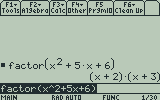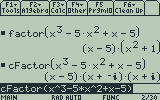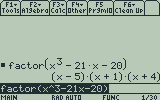# Factoring Polynomials on the TI-89 and Voyage 200

Filed under Algebra, Difficulty: Easy, TI-89, TI-92 Plus, Voyage 200.

The TI-89, TI-92 Plus, and Voyage 200 offer two built-in functions for easily factoring expressions and trinomials/polynomials into their component parts. This tutorial explains when to use these functions and how to use them to solve simple equations and polynomials.

The basic factoring function, factor(, can be reached by pressing from the home screen. To use the factor function, simply press type in your expression, add a closing parenthesis by pressing , and press .If your expression has complex roots, the factor expression may not factor it completely. To do this, press to reach the complex algebra menu and press to paste the cFactor function to the home screen. This function also breaks down factors of i, the imaginary unit. Unfortunately, it will not do this for many more complicated expressions, though these can be solved using the cSolve function.Factoring is especially useful for solving the zeroes of a polynomial equation, for example:

x³ – 21x – 20 = 0

This expression is very difficult to factor by inspection, but using the factor function, we find out that it factors into simple roots.Therefore, we know that:

(x-5)(x+1)(x+4) = 0.

By the zero product property, we know that the only way this expression can be true is if one of the individual factors is zero, which occurs when x – 5, x = -1, or x = -4. While this is not obvious looking at the original expression, factoring makes this problem easy. Factoring can also be used for more complicated expressions than simply polynomials, such as rational and trigonometric expressions.